# How to do Formula Nesting in Excel

Sometimes when working with Excel, you may need to do a calculation that involves several formulas. One way of handling this is to break each formula out in its own cell. Another more elegant solution is to nest those formulas or combine them. Let's look at an example.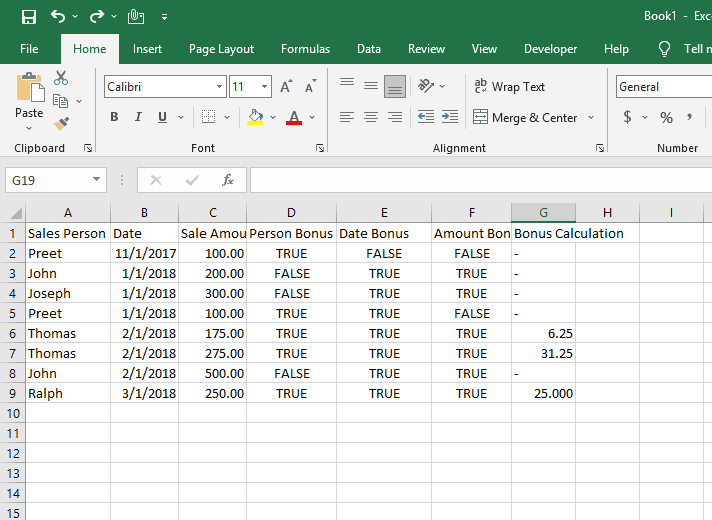The above sheet is a bonus calculator for some made-up salespeople. There are three parameters that must be met for a bonus to be paid out on a sale.

• A person must be participating in the bonus program.
• The sale must have occurred in 2018
• The amount of the sale must be greater than \$150

If all three of these criteria are met, then 25% of the sale amount over \$150 is paid out as a bonus.

## There are 4 separate formulas operating here.

• Person Bonus Calc – =OR(A2="Preet",A2="Thomas",A2="Ralph")
• This is an OR formula, which will return a TRUE if any of the expressions within the formula are true, and a FALSE if none of them are true.
• Preet, Thomas, and Ralph are participating in the bonus program. So, the formula will return a TRUE if the salesperson column has one of those three names present.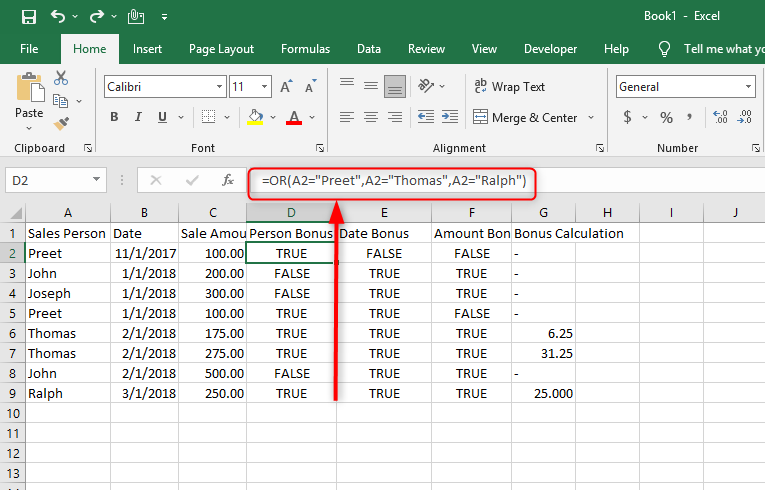• Date Bonus Calc – =YEAR(B2)>=2018
• The YEAR formula returns the year of the date in the cell it is referencing.
• Here, we are evaluating if a year of a date in the date column is greater than or equal to 2018 (the current year). If it does, the formula returns a TRUE. If not, it returns a FALSE.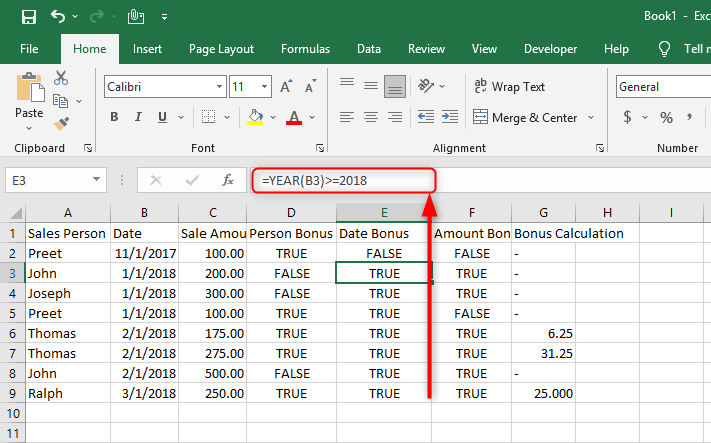• Amount Bonus Calc – =C2>150
• This is a simple expression that returns a TRUE if the sale amount in the sale amount column is greater than \$150.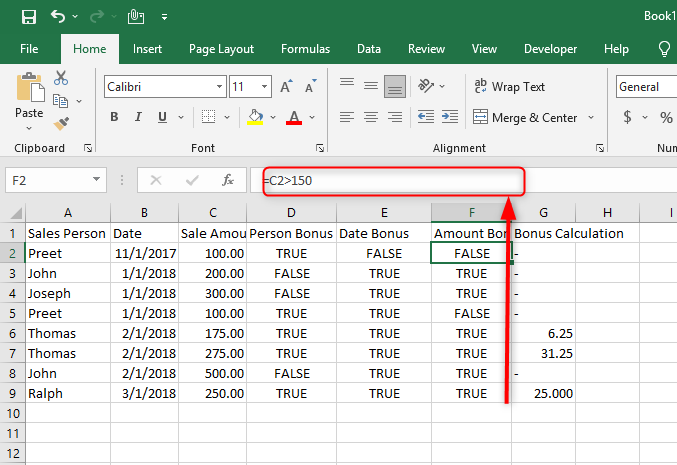• Bonus Calc – =(C6-150)*0.25
• For the sales that meet all three requirements, this formula takes the amount over \$150 and multiplies it by 25%.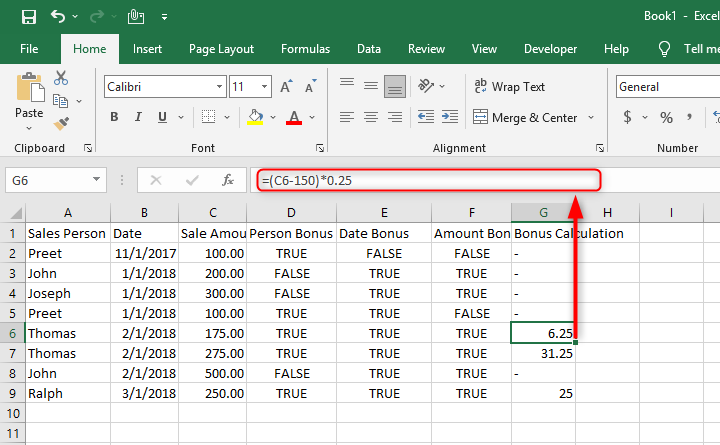Now, these formulas work and will return the results you need to figure out the bonus amounts. However, you have four columns worth of them! What if we could compress all of that into one formula. Let's look at the below, re-worked example.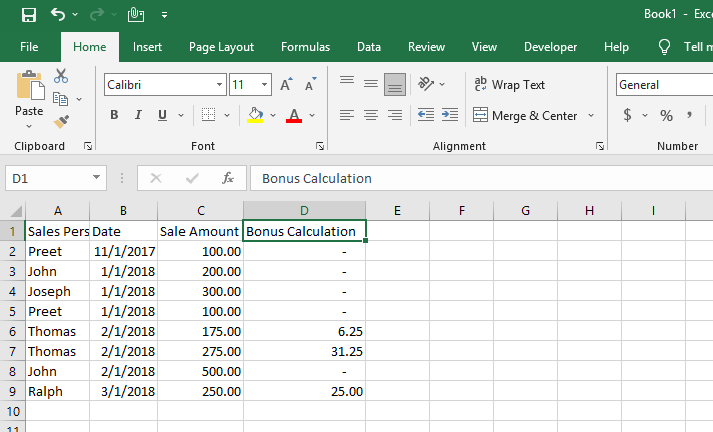The first thing you notice, all those columns are gone. We now just have one column called "Bonus Calculation". We combined all the above formulas into one nested formula. Let's explore it!

=IF(AND(OR(A2="Preet",A2="Thomas",A2="Ralph"),YEAR(B2)>=2018,C2>150),(C2-150)*0.25,0)

• First, we have an IF statement. IF statements evaluate logic you provide it, then do something based on whether that criteria returns true or false. The IF statement is going to determine if this sale qualifies for the bonus. If it does, it will do the bonus calculation, if not, it will return a 0.
• Next, there is the AND statement. This is the opposite of an OR statement in that it will only return a TRUE if every expression in the statement is TRUE. We are wrapping our three criteria for getting a bonus on a sale in this AND statement because all three of them must be true to qualify for a bonus.
• The next three formulas probably look familiar. They are the formulas we previously had broken out into three separate columns. They are now being evaluated within the AND statement.

There you have it! Instead of four columns worth of formulas, you now only have one to worry about. Nesting formulas is a great way to streamline a spreadsheet and keep the number of formulas you have to deal with to a minimum.